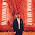### Codechef NDIFFPAL

In this post I will attempt to solve CodeChef's NDIFFPAL problem and explain my thinking as we go:

As a first observation, if we have all characters of a string identical, e.g. "aaa", we will see that there are 6 different index combo's ==> (1,1) (1,2) (1,3) (2,2) (2,3) (3,3). After some scribling around for relationship of string length and number of index combo's, this reduces easily to Triangular numbers.

Now, if we think of all the input to the puzzle that are not triangular numbers, if we reduce that number to a sum of triangular numbers that have appeared before it. e.g. 13 = 6 + 6 + 1 => so, one can represent this as "aaabbbc". The largest input possible is 10000 => closest floor triangular number to this is 9870 -- so the 140 index triangular number.

I think might as well generate all the triangular numbers till we hit the largest input and then decomposition, finally link that to printing unique strings. On second thought that it very inefficient since most of those will not be needed.

What we really need is a way to find the largest triangular number smaller than a given number and then note that and reduce the running number by this triangular number.

EDIT: An even better hack is to hard-code the 140 triangular numbers and binary search the array. This would be super-quick.

Take-aways:

1. There were much easier solutions acceptable since this is a very 'loose' problem. e.g. just repeating the character set and printing the number of character as the input would suffice as a decent base case.
2. Figurate Number are an interesting field to explore further -- this will probably re-appear a lot in problem solving!
3. Hard-coding can be king for time limits
4. ASCII to int and vice-versa

Here is the solution:

1.